This is “Classifying Chemical Reactions”, section 3.5 from the book Principles of General Chemistry (v. 1.0M). For details on it (including licensing), click here.

For more information on the source of this book, or why it is available for free, please see the project's home page. You can browse or download additional books there. To download a .zip file containing this book to use offline, simply click here.

Has this book helped you? Consider passing it on:
Creative Commons supports free culture from music to education. Their licenses helped make this book available to you.
DonorsChoose.org helps people like you help teachers fund their classroom projects, from art supplies to books to calculators.

## 3.5 Classifying Chemical Reactions

### Learning Objectives

1. To identify fundamental types of chemical reactions.
2. To predict the types of reactions substances will undergo.

The chemical reactions we have described are only a tiny sampling of the infinite number of chemical reactions possible. How do chemists cope with this overwhelming diversity? How do they predict which compounds will react with one another and what products will be formed? The key to success is to find useful ways to categorize reactions. Familiarity with a few basic types of reactions will help you to predict the products that form when certain kinds of compounds or elements come in contact.

Most chemical reactions can be classified into one or more of five basic types: acid–base reactionsA reaction of the general form acid + base → salt., exchange reactionsA chemical reaction that has the general form AB + C → AC + B or AB + CD → AD + CB., condensation reactionsA chemical reaction that has the general form A + B → AB. Condensation reactions are the reverse of cleavage reactions. Some, but not all, condensation reactions are also oxidation–reduction reactions. (and the reverse, cleavage reactionsA chemical reaction that has the general form AB → A + B. Cleavage reactions are the reverse of condensation reactions.), and oxidation–reduction reactionsA chemical reaction that exhibits a change in the oxidation states of one or more elements in the reactants that has the general form oxidant + reductant → reduced oxidant + oxidized reductant.. The general forms of these five kinds of reactions are summarized in Table 3.1 "Basic Types of Chemical Reactions", along with examples of each. It is important to note, however, that many reactions can be assigned to more than one classification, as you will see in our discussion. The classification scheme is only for convenience; the same reaction can be classified in different ways, depending on which of its characteristics is most important. Oxidation–reduction reactions, in which there is a net transfer of electrons from one atom to another, and condensation reactions are discussed in this section. Acid–base reactions and one kind of exchange reaction—the formation of an insoluble salt such as barium sulfate when solutions of two soluble salts are mixed together—will be discussed in Chapter 4 "Reactions in Aqueous Solution".

Table 3.1 Basic Types of Chemical Reactions

Name of Reaction General Form Example(s)
oxidation–reduction (redox) oxidant + reductant → reduced oxidant + oxidized reductant C7H16(l) + 11O2(g) → 7CO2(g) + 8H2O(g)
acid–base acid + base → salt NH3(aq) + HNO3(aq) → NH4+(aq) + NO3(aq)
exchange AB + C → AC + B CH3Cl + OH → CH3OH + Cl
AB + CD → AD + CB BaCl2(aq) + Na2SO4(aq) → BaSO4(s) + 2NaCl(aq)
condensation A + B → AB CO2(g) + H2O(l) → H2CO3(aq)
HBr + H2C=CH2 → CH3CH2Br*
cleavage AB → A + B CaCO3(s) → CaO(s) + CO2(g)
CH3CH2Cl → H2C=CH2 + HCl**
* In more advanced chemisty courses you will learn that this reaction is also called an addition reaction.
** In more advanced chemistry courses you will learn that this reaction is also called an elimination reaction.

## Oxidation–Reduction Reactions

The term oxidationThe loss of one or more electrons in a chemical reaction. The substance that loses electrons is said to be oxidized. was first used to describe reactions in which metals react with oxygen in air to produce metal oxides. When iron is exposed to air in the presence of water, for example, the iron turns to rust—an iron oxide. When exposed to air, aluminum metal develops a continuous, coherent, transparent layer of aluminum oxide on its surface. In both cases, the metal acquires a positive charge by transferring electrons to the neutral oxygen atoms of an oxygen molecule. As a result, the oxygen atoms acquire a negative charge and form oxide ions (O2−). Because the metals have lost electrons to oxygen, they have been oxidized; oxidation is therefore the loss of electrons. Conversely, because the oxygen atoms have gained electrons, they have been reduced, so reduction is the gain of electrons. For every oxidation, there must be an associated reduction.

### Note the Pattern

Any oxidation must be accompanied by a reduction and vice versa.

Originally, the term reductionThe gain of one or more electrons in a chemical reaction. The substance that gains electrons is said to be reduced. referred to the decrease in mass observed when a metal oxide was heated with carbon monoxide, a reaction that was widely used to extract metals from their ores. When solid copper(I) oxide is heated with hydrogen, for example, its mass decreases because the formation of pure copper is accompanied by the loss of oxygen atoms as a volatile product (water). The reaction is as follows:

Equation 3.24

Cu2O(s) + H2(g) → 2Cu(s) + H2O(g)

Oxidation and reduction reactions are now defined as reactions that exhibit a change in the oxidation states of one or more elements in the reactants, which follows the mnemonic oxidation is loss reduction is gain, or oil rig. The oxidation stateThe charge that each atom in a compound would have if all its bonding electrons were transferred to the atom with the greater attraction for electrons. of each atom in a compound is the charge an atom would have if all its bonding electrons were transferred to the atom with the greater attraction for electrons. Atoms in their elemental form, such as O2 or H2, are assigned an oxidation state of zero. For example, the reaction of aluminum with oxygen to produce aluminum oxide is

Equation 3.25

4Al(s) + 3O2(g) → 2Al2O3(s)

Each neutral oxygen atom gains two electrons and becomes negatively charged, forming an oxide ion; thus, oxygen has an oxidation state of −2 in the product and has been reduced. Each neutral aluminum atom loses three electrons to produce an aluminum ion with an oxidation state of +3 in the product, so aluminum has been oxidized. In the formation of Al2O3, electrons are transferred as follows (the superscript 0 emphasizes the oxidation state of the elements):

Equation 3.26

4Al0 + 3O20 → 4Al3+ + 6O2−

Equation 3.24 and Equation 3.25 are examples of oxidation–reduction (redox) reactions. In redox reactions, there is a net transfer of electrons from one reactant to another. In any redox reaction, the total number of electrons lost must equal the total of electrons gained to preserve electrical neutrality. In Equation 3.26, for example, the total number of electrons lost by aluminum is equal to the total number gained by oxygen:

Equation 3.27

The same pattern is seen in all oxidation–reduction reactions: the number of electrons lost must equal the number of electrons gained.

An additional example of a redox reaction, the reaction of sodium metal with oxygen in air, is illustrated in Figure 3.1 "Samples of 1 Mol of Some Common Substances".

### Note the Pattern

In all oxidation–reduction (redox) reactions, the number of electrons lost equals the number of electrons gained.

## Assigning Oxidation States

Assigning oxidation states to the elements in binary ionic compounds is straightforward: the oxidation states of the elements are identical to the charges on the monatomic ions. In Chapter 2 "Molecules, Ions, and Chemical Formulas", you learned how to predict the formulas of simple ionic compounds based on the sign and magnitude of the charge on monatomic ions formed by the neutral elements. Examples of such compounds are sodium chloride (NaCl; Figure 3.13 "The Reaction of a Neutral Sodium Atom with a Neutral Chlorine Atom"), magnesium oxide (MgO), and calcium chloride (CaCl2). In covalent compounds, in contrast, atoms share electrons. Oxidation states in covalent compounds are somewhat arbitrary, but they are useful bookkeeping devices to help you understand and predict many reactions.

Figure 3.13 The Reaction of a Neutral Sodium Atom with a Neutral Chlorine Atom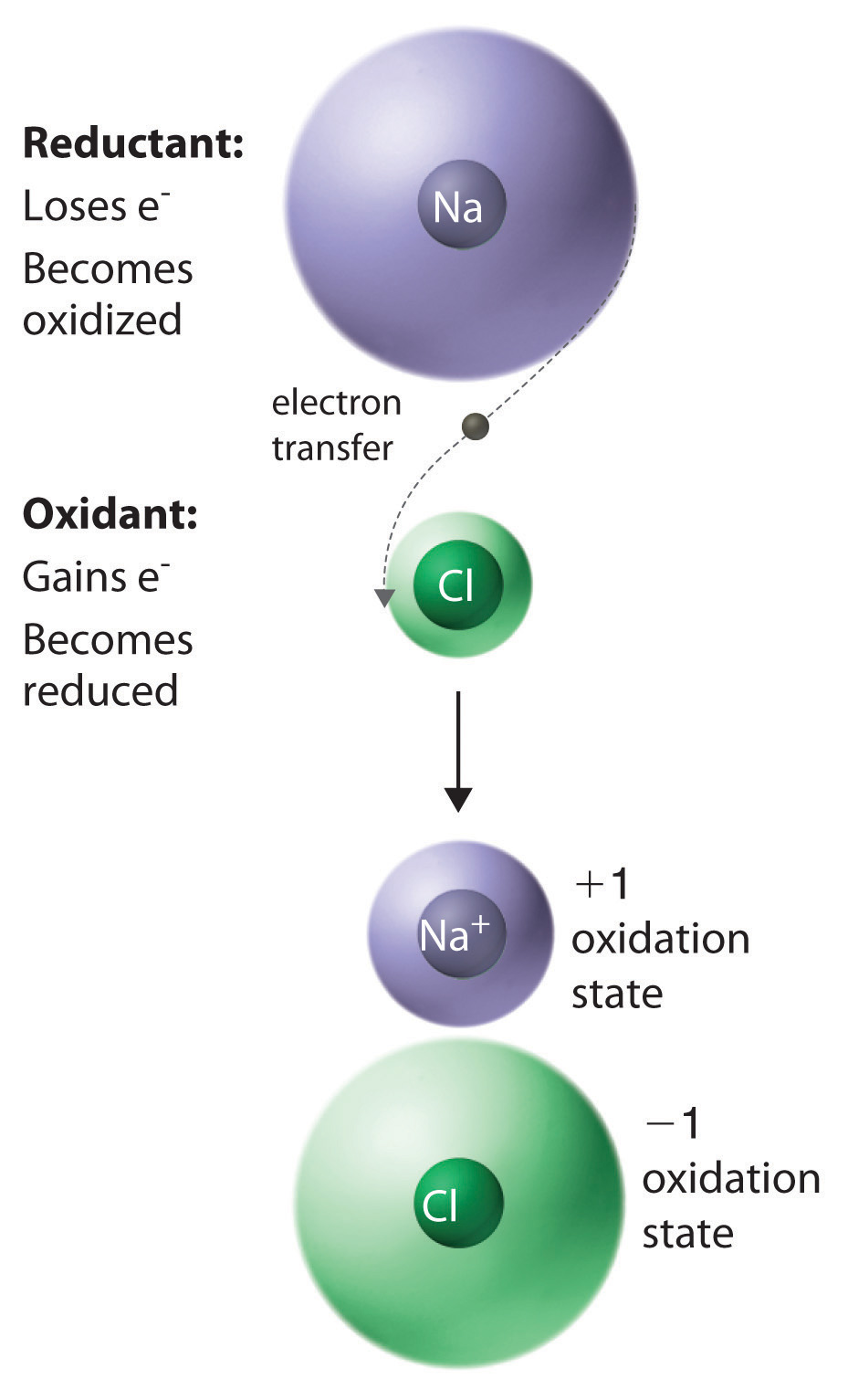The result is the transfer of one electron from sodium to chlorine, forming the ionic compound NaCl.

A set of rules for assigning oxidation states to atoms in chemical compounds follows. As we discuss atomic and molecular structure in Chapter 6 "The Structure of Atoms", Chapter 7 "The Periodic Table and Periodic Trends", Chapter 8 "Ionic versus Covalent Bonding", and Chapter 9 "Molecular Geometry and Covalent Bonding Models", the principles underlying these rules will be described more fully.

## Rules for Assigning Oxidation StatesNonintegral oxidation states are encountered occasionally. They are usually due to the presence of two or more atoms of the same element with different oxidation states.

1. The oxidation state of an atom in any pure element, whether monatomic, diatomic, or polyatomic, is zero.
2. The oxidation state of a monatomic ion is the same as its charge—for example, Na+ = +1, Cl = −1.
3. The oxidation state of fluorine in chemical compounds is always −1. Other halogens usually have oxidation states of −1 as well, except when combined with oxygen or other halogens.
4. Hydrogen is assigned an oxidation state of +1 in its compounds with nonmetals and −1 in its compounds with metals.
5. Oxygen is normally assigned an oxidation state of −2 in compounds, with two exceptions: in compounds that contain oxygen–fluorine or oxygen–oxygen bonds, the oxidation state of oxygen is determined by the oxidation states of the other elements present.
6. The sum of the oxidation states of all the atoms in a neutral molecule or ion must equal the charge on the molecule or ion.

In any chemical reaction, the net charge must be conserved; that is, in a chemical reaction, the total number of electrons is constant, just like the total number of atoms. Consistent with this, rule 1 states that the sum of the individual oxidation states of the atoms in a molecule or ion must equal the net charge on that molecule or ion. In NaCl, for example, Na has an oxidation state of +1 and Cl is −1. The net charge is zero, as it must be for any compound.

Rule 3 is required because fluorine attracts electrons more strongly than any other element, for reasons you will discover in Chapter 6 "The Structure of Atoms". Hence fluorine provides a reference for calculating the oxidation states of other atoms in chemical compounds. Rule 4 reflects the difference in chemistry observed for compounds of hydrogen with nonmetals (such as chlorine) as opposed to compounds of hydrogen with metals (such as sodium). For example, NaH contains the H ion, whereas HCl forms H+ and Cl ions when dissolved in water. Rule 5 is necessary because fluorine has a greater attraction for electrons than oxygen does; this rule also prevents violations of rule 2. So the oxidation state of oxygen is +2 in OF2 but −½ in KO2. Note that an oxidation state of −½ for O in KO2 is perfectly acceptable.

The reduction of copper(I) oxide shown in Equation 3.28 demonstrates how to apply these rules. Rule 1 states that atoms in their elemental form have an oxidation state of zero, which applies to H2 and Cu. From rule 4, hydrogen in H2O has an oxidation state of +1, and from rule 5, oxygen in both Cu2O and H2O has an oxidation state of −2. Rule 6 states that the sum of the oxidation states in a molecule or formula unit must equal the net charge on that compound. This means that each Cu atom in Cu2O must have a charge of +1: 2(+1) + (−2) = 0. So the oxidation states are as follows:

Equation 3.28

$Cu 2 +1 O –2 (s) + H 2 0 (g) → 2 Cu 0 (s) + H 2 +1 O –2 (g)$

Assigning oxidation states allows us to see that there has been a net transfer of electrons from hydrogen (0 → +1) to copper (+1 → 0). So this is a redox reaction. Once again, the number of electrons lost equals the number of electrons gained, and there is a net conservation of charge:

Equation 3.29

Remember that oxidation states are useful for visualizing the transfer of electrons in oxidation–reduction reactions, but the oxidation state of an atom and its actual charge are the same only for simple ionic compounds. Oxidation states are a convenient way of assigning electrons to atoms, and they are useful for predicting the types of reactions that substances undergo.

### Example 14

Assign oxidation states to all atoms in each compound.

1. sulfur hexafluoride (SF6)
2. methanol (CH3OH)
3. ammonium sulfate [(NH4)2SO4]
4. magnetite (Fe3O4)
5. ethanoic (acetic) acid (CH3CO2H)

Given: molecular or empirical formula

Asked for: oxidation states

Strategy:

Begin with atoms whose oxidation states can be determined unambiguously from the rules presented (such as fluorine, other halogens, oxygen, and monatomic ions). Then determine the oxidation states of other atoms present according to rule 1.

Solution:

1. We know from rule 3 that fluorine always has an oxidation state of −1 in its compounds. The six fluorine atoms in sulfur hexafluoride give a total negative charge of −6. Because rule 1 requires that the sum of the oxidation states of all atoms be zero in a neutral molecule (here SF6), the oxidation state of sulfur must be +6:

[(6 F atoms)(−1)] + [(1 S atom) (+6)] = 0
2. According to rules 4 and 5, hydrogen and oxygen have oxidation states of +1 and −2, respectively. Because methanol has no net charge, carbon must have an oxidation state of −2:

[(4 H atoms)(+1)] + [(1 O atom)(−2)] + [(1 C atom)(−2)] = 0
3. Note that (NH4)2SO4 is an ionic compound that consists of both a polyatomic cation (NH4+) and a polyatomic anion (SO42−) (see Table 2.4 "Common Polyatomic Ions and Their Names"). We assign oxidation states to the atoms in each polyatomic ion separately. For NH4+, hydrogen has an oxidation state of +1 (rule 4), so nitrogen must have an oxidation state of −3:

[(4 H atoms)(+1)] + [(1 N atom)(−3)] = +1, the charge on the NH4+ ion

For SO42−, oxygen has an oxidation state of −2 (rule 5), so sulfur must have an oxidation state of +6:

[(4 O atoms) (−2)] + [(1 S atom)(+6)] = −2, the charge on the sulfate ion
4. Oxygen has an oxidation state of −2 (rule 5), giving an overall charge of −8 per formula unit. This must be balanced by the positive charge on three iron atoms, giving an oxidation state of +8/3 for iron:

Fractional oxidation states are allowed because oxidation states are a somewhat arbitrary way of keeping track of electrons. In fact, Fe3O4 can be viewed as having two Fe3+ ions and one Fe2+ ion per formula unit, giving a net positive charge of +8 per formula unit. Fe3O4 is a magnetic iron ore commonly called magnetite. In ancient times, magnetite was known as lodestone because it could be used to make primitive compasses that pointed toward Polaris (the North Star), which was called the “lodestar.”

5. Initially, we assign oxidation states to the components of CH3CO2H in the same way as any other compound. Hydrogen and oxygen have oxidation states of +1 and −2 (rules 4 and 5, respectively), resulting in a total charge for hydrogen and oxygen of

[(4 H atoms)(+1)] + [(2 O atoms)(−2)] = 0

So the oxidation state of carbon must also be zero (rule 6). This is, however, an average oxidation state for the two carbon atoms present. Because each carbon atom has a different set of atoms bonded to it, they are likely to have different oxidation states. To determine the oxidation states of the individual carbon atoms, we use the same rules as before but with the additional assumption that bonds between atoms of the same element do not affect the oxidation states of those atoms. The carbon atom of the methyl group (−CH3) is bonded to three hydrogen atoms and one carbon atom. We know from rule 4 that hydrogen has an oxidation state of +1, and we have just said that the carbon–carbon bond can be ignored in calculating the oxidation state of the carbon atom. For the methyl group to be electrically neutral, its carbon atom must have an oxidation state of −3. Similarly, the carbon atom of the carboxylic acid group (−CO2H) is bonded to one carbon atom and two oxygen atoms. Again ignoring the bonded carbon atom, we assign oxidation states of −2 and +1 to the oxygen and hydrogen atoms, respectively, leading to a net charge of

[(2 O atoms)(−2)] + [(1 H atom)(+1)] = −3

To obtain an electrically neutral carboxylic acid group, the charge on this carbon must be +3. The oxidation states of the individual atoms in acetic acid are thus

$C − 3 H 3 + 1 C + 3 O 2 − 2 H + 1$

Thus the sum of the oxidation states of the two carbon atoms is indeed zero.

Exercise

Assign oxidation states to all atoms in each compound.

1. barium fluoride (BaF2)
2. formaldehyde (CH2O)
3. potassium dichromate (K2Cr2O7)
4. cesium oxide (CsO2)
5. ethanol (CH3CH2OH)

Answer:

1. Ba, +2; F, −1
2. C, 0; H, +1; O, −2
3. K, +1; Cr, +6; O, −2
4. Cs, +1; O, −½
5. C, −3; H, +1; C, −1; H, +1; O, −2; H, +1

## Oxidants and Reductants

Compounds that are capable of accepting electrons, such as O2 or F2, are called oxidants (or oxidizing agents)A compound that is capable of accepting electrons; thus it is reduced. because they can oxidize other compounds. In the process of accepting electrons, an oxidant is reduced. Compounds that are capable of donating electrons, such as sodium metal or cyclohexane (C6H12), are called reductants (or reducing agents)A compound that is capable of donating electrons; thus it is oxidized. because they can cause the reduction of another compound. In the process of donating electrons, a reductant is oxidized. These relationships are summarized in Equation 3.30:

Equation 3.30

oxidant + reductant → oxidation−reduction

Some oxidants have a greater ability than others to remove electrons from other compounds. Oxidants can range from very powerful, capable of oxidizing most compounds with which they come in contact, to rather weak. Both F2 and Cl2 are powerful oxidants: for example, F2 will oxidize H2O in a vigorous, potentially explosive reaction. In contrast, S8 is a rather weak oxidant, and O2 falls somewhere in between. Conversely, reductants vary in their tendency to donate electrons to other compounds. Reductants can also range from very powerful, capable of giving up electrons to almost anything, to weak. The alkali metals are powerful reductants, so they must be kept away from atmospheric oxygen to avoid a potentially hazardous redox reaction.

A combustion reactionAn oxidation–reduction reaction in which the oxidant is $O2$., first introduced in Section 3.2 "Determining Empirical and Molecular Formulas", is an oxidation–reduction reaction in which the oxidant is O2. One example of a combustion reaction is the burning of a candle, shown in Figure 3.9 "An Example of a Combustion Reaction". Consider, for example, the combustion of cyclohexane, a typical hydrocarbon, in excess oxygen. The balanced chemical equation for the reaction, with the oxidation state shown for each atom, is as follows:

Equation 3.31

$C 6 –2 H 12 +1 + 9O 2 0 → 6 C +4 O 2 –2 + 6 H 2 +1 O –2$

If we compare the oxidation state of each element in the products and the reactants, we see that hydrogen is the only element whose oxidation state does not change; it remains +1. Carbon, however, has an oxidation state of −2 in cyclohexane and +4 in CO2; that is, each carbon atom changes its oxidation state by six electrons during the reaction. Oxygen has an oxidation state of 0 in the reactants, but it gains electrons to have an oxidation state of −2 in CO2 and H2O. Because carbon has been oxidized, cyclohexane is the reductant; because oxygen has been reduced, it is the oxidant. All combustion reactions are therefore oxidation–reduction reactions.

## Condensation Reactions

The reaction of bromine with ethylene to give 1,2-dibromoethane, which is used in agriculture to kill nematodes in soil, is as follows:

Equation 3.32

C2H4(g) + Br2(g) → BrCH2CH2Br(g)

According to Table 3.1 "Basic Types of Chemical Reactions", this is a condensation reaction because it has the general form A + B → AB. This reaction, however, can also be viewed as an oxidation–reduction reaction, in which electrons are transferred from carbon (−2 → −1) to bromine (0 → −1). Another example of a condensation reaction is the one used for the industrial synthesis of ammonia:

Equation 3.33

3H2(g) + N2(g) → 2NH3(g)

Although this reaction also has the general form of a condensation reaction, hydrogen has been oxidized (0 → +1) and nitrogen has been reduced (0 → −3), so it can also be classified as an oxidation–reduction reaction.

Not all condensation reactions are redox reactions. The reaction of an amine with a carboxylic acid, for example, is a variant of a condensation reaction (A + B → A′B′ + C): two large fragments condense to form a single molecule, and a much smaller molecule, such as H2O, is eliminated. In this reaction, the −OH from the carboxylic acid group and −H from the amine group are eliminated as H2O, and the reaction forms an amide bond (also called a peptide bond) that links the two fragments. Amide bonds are the essential structural unit linking the building blocks of proteins and many polymers together, as described in Chapter 12 "Solids". Nylon, for example, is produced from a condensation reaction (Figure 3.14 "The Production of Nylon").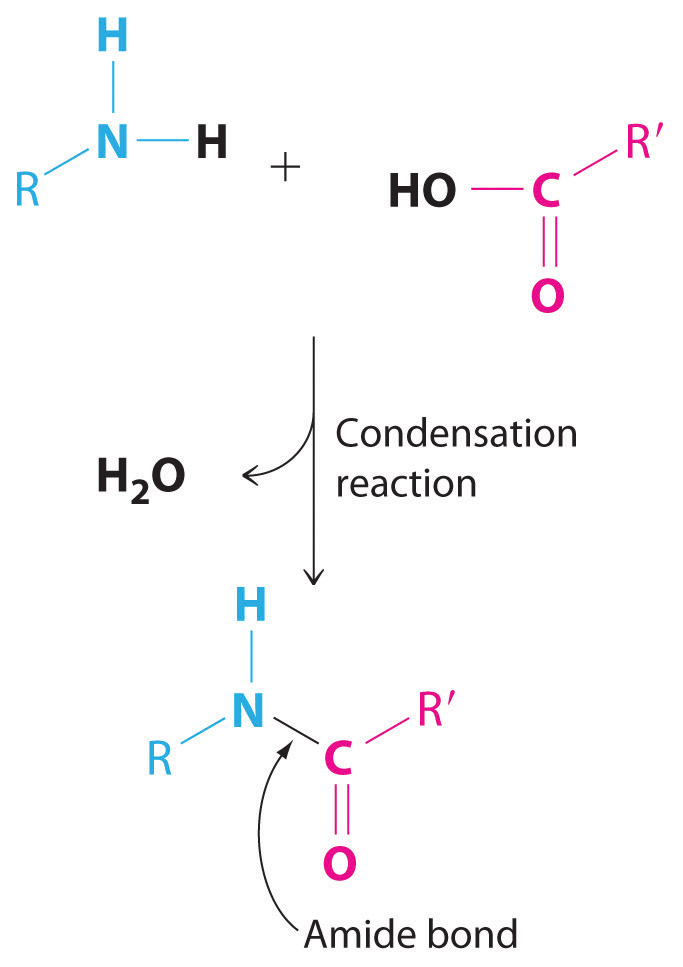Amide bonds. The reaction of an amine with a carboxylic acid proceeds by eliminating water and forms a new C–N (amide) bond.

Figure 3.14 The Production of Nylon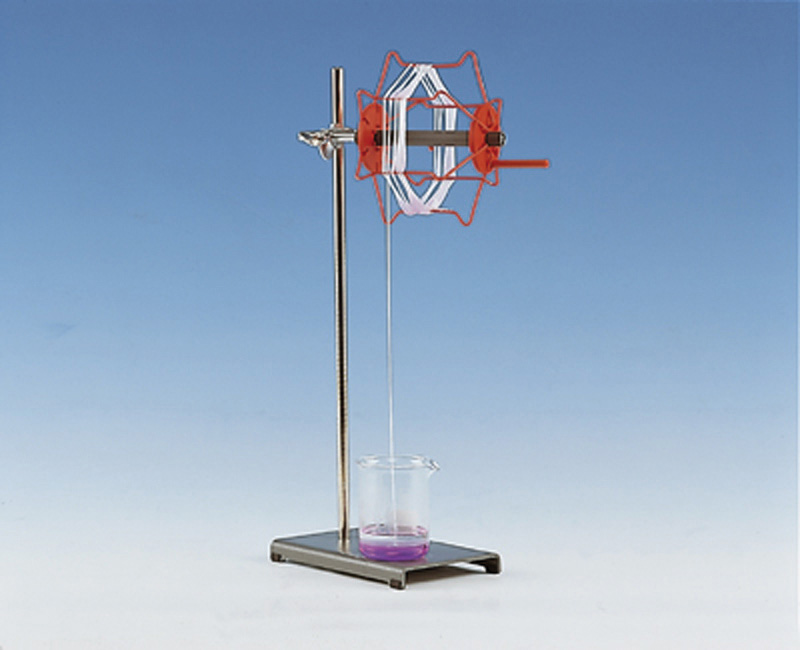### Example 15

The following reactions have important industrial applications. Using Table 3.1 "Basic Types of Chemical Reactions", classify each reaction as an oxidation–reduction reaction, an acid–base reaction, an exchange reaction, a condensation reaction, or a cleavage reaction. For each redox reaction, identify the oxidant and reductant and specify which atoms are oxidized or reduced. (Don’t forget that some reactions can be placed into more than one category.)

1. C2H4(g) + Cl2(g) → ClCH2CH2Cl(g)
2. AgNO3(aq) + NaCl(aq) → AgCl(s) + NaNO3(aq)
3. CaCO3(s) → CaO(s) + CO2(g)
4. Ca5(PO4)3(OH)(s) + 7H3PO4(aq) + 4H2O(l) → 5Ca(H2PO4)2·H2O(s)
5. Pb(s) + PbO2(s) + 2H2SO4(aq) → 2PbSO4(s) + 2H2O(l)

Given: balanced chemical equation

Asked for: classification of chemical reaction

Strategy:

A Determine the general form of the equation by referring to Table 3.1 "Basic Types of Chemical Reactions" and then classify the reaction.

B For redox reactions, assign oxidation states to each atom present in the reactants and the products. If the oxidation state of one or more atoms changes, then the reaction is a redox reaction. If not, the reaction must be one of the other types of reaction listed in Table 3.1 "Basic Types of Chemical Reactions".

Solution:

1. A This reaction is used to prepare 1,2-dichloroethane, one of the top 25 industrial chemicals in Figure 2.22 "Top 25 Chemicals Produced in the United States in 2002*". It has the general form A + B → AB, which is typical of a condensation reaction. B Because reactions may fit into more than one category, we need to look at the oxidation states of the atoms:

$C 2 –2 H 4 +1 + Cl 2 0 → Cl –1 C –1 H 2 +1 C –1 H 2 +1 Cl –1$

The oxidation states show that chlorine is reduced from 0 to −1 and carbon is oxidized from −2 to −1, so this is a redox reaction as well as a condensation reaction. Ethylene is the reductant, and chlorine is the oxidant.

2. A This reaction is used to prepare silver chloride for making photographic film. The chemical equation has the general form AB + CD → AD + CB, so it is classified as an exchange reaction. B The oxidation states of the atoms are as follows

$Ag +1 N +5 O 3 –2 + Na +1 Cl –1 → Ag +1 Cl –1 + Na +1 N +5 O 3 –2$

There is no change in the oxidation states, so this is not a redox reaction.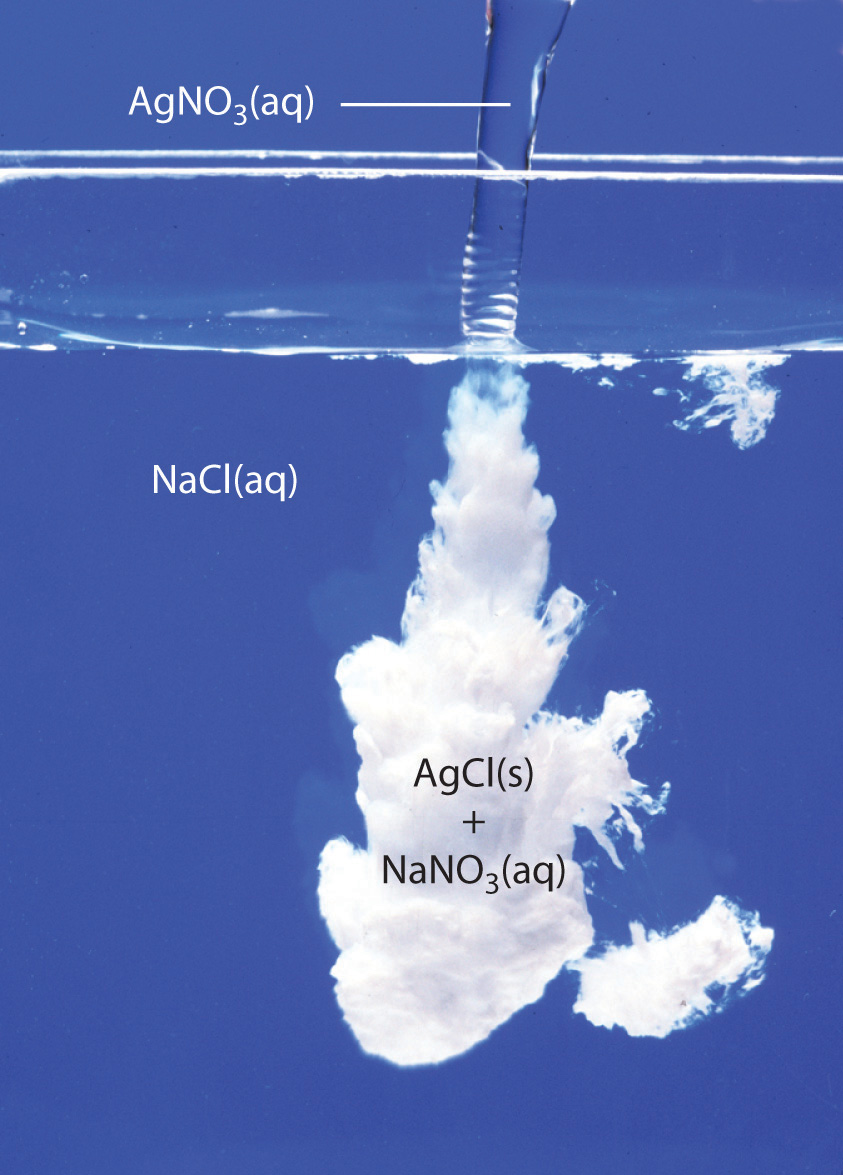AgCl(s) precipitates when solutions of AgNO3(aq) and NaCl(aq) are mixed. NaNO3 (aq) is in solution as Na+ and NO3 ions.

3. A This reaction is used to prepare lime (CaO) from limestone (CaCO3) and has the general form AB → A + B. The chemical equation’s general form indicates that it can be classified as a cleavage reaction, the reverse of a condensation reaction. B The oxidation states of the atoms are as follows:

$Ca +2 C +4 O 3 –2 → Ca +2 O –2 + C +4 O 2 –2$

Because the oxidation states of all the atoms are the same in the products and the reactant, this is not a redox reaction.

4. A This reaction is used to prepare “super triple phosphate” in fertilizer. One of the reactants is phosphoric acid, which transfers a proton (H+) to the phosphate and hydroxide ions of hydroxyapatite [Ca5(PO4)3(OH)] to form H2PO4 and H2O, respectively. This is an acid–base reaction, in which H3PO4 is the acid (H+ donor) and Ca5(PO4)3(OH) is the base (H+ acceptor).

B To determine whether it is also a redox reaction, we assign oxidation states to the atoms:

$Ca 5 +2 (P +5 O 4 –2 ) 3 ( O –2 H +1 ) + 7H 3 +1 P +5 O 4 –2 + 4 H +1 2 O –2 → 5 Ca +2 ( H +1 2 P +5 O 4 –2 ) 2 · H 2 + 1 O –2$

Because there is no change in oxidation state, this is not a redox reaction.

5. A This reaction occurs in a conventional car battery every time the engine is started. An acid (H2SO4) is present and transfers protons to oxygen in PbO2 to form water during the reaction. The reaction can therefore be described as an acid–base reaction.

B The oxidation states are as follows:

$Pb 0 + Pb +4 O 2 –2 + 2 H 2 +1 S +6 O 4 –2 → 2 Pb +2 S +6 O 4 –2 + 2 H 2 +1 O –2$

The oxidation state of lead changes from 0 in Pb and +4 in PbO2 (both reactants) to +2 in PbSO4. This is also a redox reaction, in which elemental lead is the reductant, and PbO2 is the oxidant. Which description is correct? Both.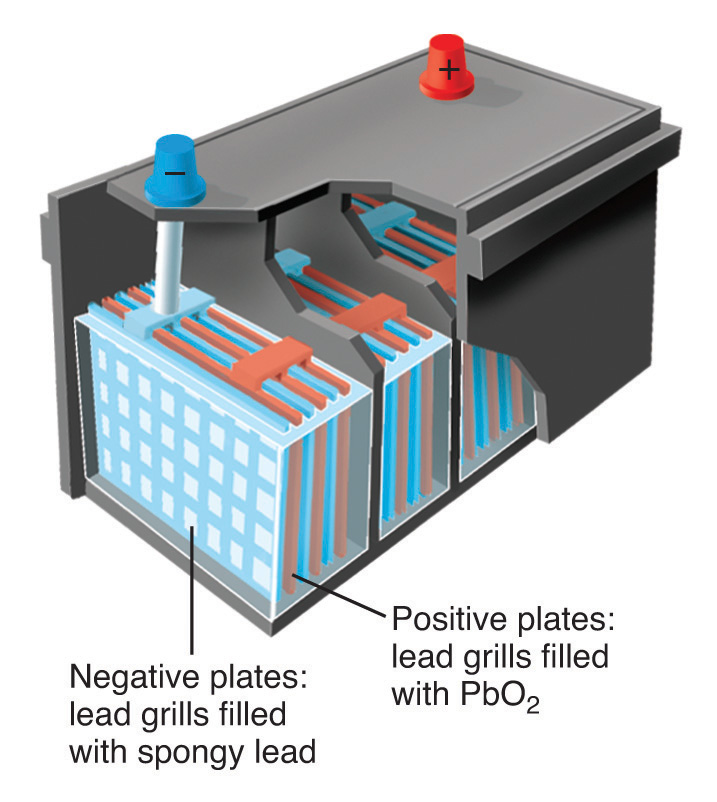Schematic drawing of a 12-volt car battery. The locations of the reactants (lead metal in a spongy form with large surface area) and PbO2 are shown. The product (PbSO4) forms as a white solid between the plates.

Exercise

Using Table 3.1 "Basic Types of Chemical Reactions", classify each reaction as an oxidation–reduction reaction, an acid–base reaction, an exchange reaction, a condensation reaction, or a cleavage reaction. For each redox reaction, identify the oxidant and the reductant and specify which atoms are oxidized or reduced.

1. Al(s) + OH(aq) + 3H2O(l) → 3/2H2(g) + [Al(OH)4](aq)
2. TiCl4(l) + 2Mg(l) → Ti(s) + 2MgCl2(l)
3. MgCl2(aq) + Na2CO3(aq) → MgCO3(s) + 2NaCl(aq)
4. CO(g) + Cl2(g) → Cl2CO(l)
5. H2SO4(l) + 2NH3(g) → (NH4)2SO4(s)

Answer:

1. Redox reaction; reductant is Al, oxidant is H2O; Al is oxidized, H is reduced. This is the reaction that occurs when Drano is used to clear a clogged drain.
2. Redox reaction; reductant is Mg, oxidant is TiCl4; Mg is oxidized, Ti is reduced.
3. Exchange reaction. This reaction is responsible for the scale that develops in coffee makers in areas that have hard water.
4. Both a condensation reaction and a redox reaction; reductant is CO, oxidant is Cl2; C is oxidized, Cl is reduced. The product of this reaction is phosgene, a highly toxic gas used as a chemical weapon in World War I. Phosgene is now used to prepare polyurethanes, which are used in foams for bedding and furniture and in a variety of coatings.
5. Acid–base reaction.

## Catalysts

Many chemical reactions, including some of those discussed previously, occur more rapidly in the presence of a catalystA substance that increases the rate of a chemical reaction without undergoing a net chemical change itself., which is a substance that participates in a reaction and causes it to occur more rapidly but can be recovered unchanged at the end of a reaction and reused. Because catalysts are not involved in the stoichiometry of a reaction, they are usually shown above the arrow in a net chemical equation. Chemical processes in industry rely heavily on the use of catalysts, which are usually added to a reaction mixture in trace amounts, and most biological reactions do not take place without a biological catalyst or enzymeCatalysts that occur naturally in living organisms and catalyze biological reactions.. Examples of catalyzed reactions in industry are the use of platinum in petroleum cracking and reforming, the reaction of SO2 and O2 in the presence of V2O5 to produce SO3 in the industrial synthesis of sulfuric acid, and the use of sulfuric acid in the synthesis of compounds such as ethyl acetate and procaine. Not only do catalysts greatly increase the rates of reactions, but in some cases such as in petroleum refining, they also control which products are formed. The acceleration of a reaction by a catalyst is called catalysisThe acceleration of a chemical reaction by a catalyst..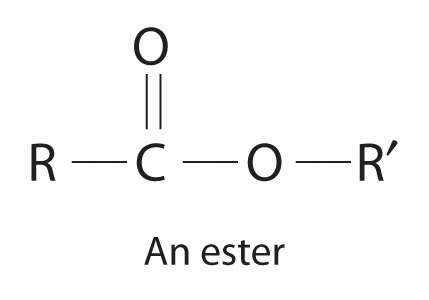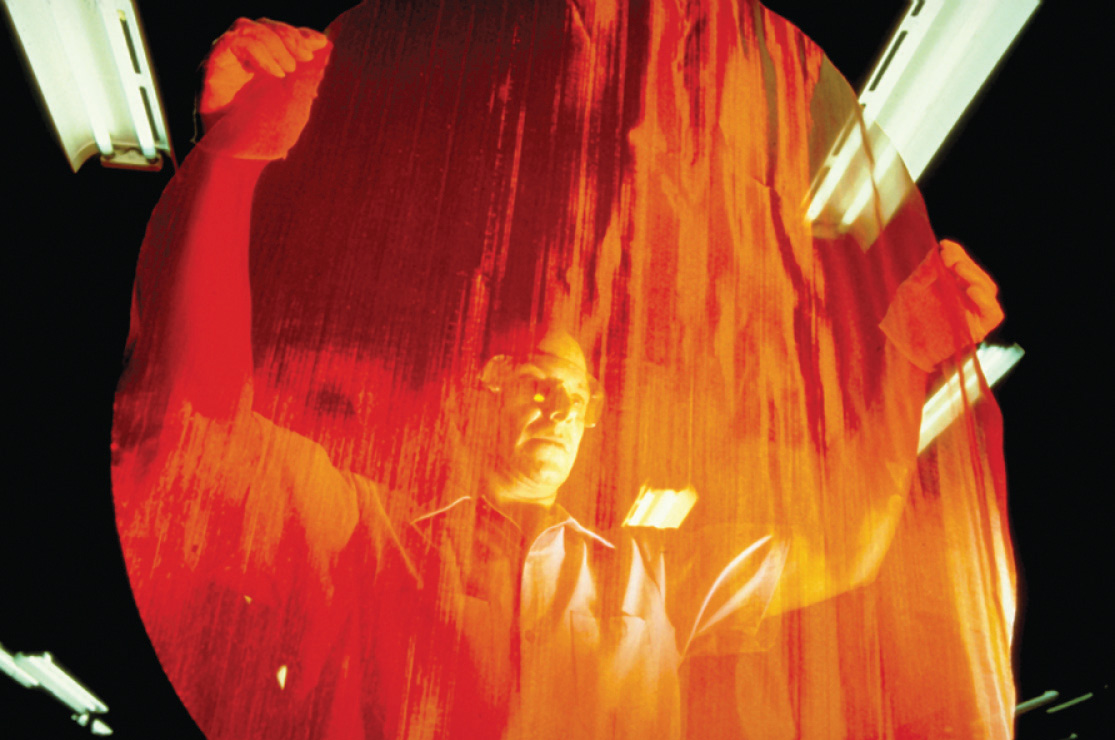A heterogeneous catalyst. This large circular gauze, woven from rhodium-platinum wire, is a heterogeneous catalyst in the commercial production of nitric acid by the oxidation of ammonia.

Catalysts may be classified as either homogeneous or heterogeneous. A homogeneous catalystA catalyst that is uniformly dispersed throughout the reactant mixture to form a solution. is uniformly dispersed throughout the reactant mixture to form a solution. Sulfuric acid, for example, is a homogeneous catalyst used in the synthesis of esters such as procaine (Example 13). An ester has a structure similar to that of a carboxylic acid, in which the hydrogen atom attached to oxygen has been replaced by an R group. They are responsible for the fragrances of many fruits, flowers, and perfumes. Other examples of homogeneous catalysts are the enzymes that allow our bodies to function. In contrast, a heterogeneous catalystA catalyst that is in a different physical state than the reactants. is in a different physical state than the reactants. For economic reasons, most industrial processes use heterogeneous catalysts in the form of solids that are added to solutions of the reactants. Because such catalysts often contain expensive precious metals such as platinum or palladium, it makes sense to formulate them as solids that can be easily separated from the liquid or gaseous reactant-product mixture and recovered. Examples of heterogeneous catalysts are the iron oxides used in the industrial synthesis of ammonia and the catalytic converters found in virtually all modern automobiles, which contain precious metals like palladium and rhodium. Catalysis will be discussed in more detail in Chapter 14 "Chemical Kinetics" when we discuss reaction rates, but you will encounter the term frequently throughout the text.

### Summary

Chemical reactions may be classified as an acid–base reaction, an exchange reaction, a condensation reaction and its reverse, a cleavage reaction, and an oxidation–reduction (or redox) reaction. To keep track of electrons in chemical reactions, oxidation states are assigned to atoms in compounds. The oxidation state is the charge an atom would have if all its bonding electrons were transferred completely to the atom that has the greater attraction for electrons. In an oxidation–reduction reaction, one atom must lose electrons and another must gain electrons. Oxidation is the loss of electrons, and an element whose oxidation state increases is said to be oxidized. Reduction is the gain of electrons, and an element whose oxidation state decreases is said to be reduced. Oxidants are compounds that are capable of accepting electrons from other compounds, so they are reduced during an oxidation–reduction reaction. In contrast, reductants are compounds that are capable of donating electrons to other compounds, so they are oxidized during an oxidation–reduction reaction. A combustion reaction is a redox reaction in which the oxidant is O2(g). An amide bond is formed from the condensation reaction between a carboxylic acid and an amine; it is the essential structural unit of proteins and many polymers. A catalyst is a substance that increases the rate of a chemical reaction without undergoing a net chemical change itself. A biological catalyst is called an enzyme. Catalysis is an acceleration in the rate of a reaction caused by the presence of a substance that does not appear in the chemical equation. A homogeneous catalyst is uniformly dispersed in a solution of the reactants, whereas a heterogeneous catalyst is present as a different phase, usually a solid.

### Key Takeaway

• Chemical reactions may be classified as acid–base, exchange, condensation, cleavage, and oxidation–reduction (redox).

### Conceptual Problems

1. What is a combustion reaction? How can it be distinguished from an exchange reaction?

2. What two products are formed in the combustion of an organic compound containing only carbon, hydrogen, and oxygen? Is it possible to form only these two products from a reaction that is not a combustion reaction? Explain your answer.

3. What factors determine whether a reaction can be classified as a redox reaction?

4. Name three characteristics of a balanced redox reaction.

5. Does an oxidant accept electrons or donate them?

6. Does the oxidation state of a reductant become more positive or more negative during a redox reaction?

7. Nitrogen, hydrogen, and ammonia are known to have existed on primordial earth, yet mixtures of nitrogen and hydrogen do not usually react to give ammonia. What natural phenomenon would have enough energy to initiate a reaction between these two primordial gases?

8. Catalysts are not added to reactions in stoichiometric quantities. Why?

9. State whether each of the following uses a homogeneous catalyst or a heterogeneous catalyst.

1. Platinum metal is used in the catalytic converter of an automobile.
2. Nitrogen is biologically converted to ammonia by an enzyme.
3. Carbon monoxide and hydrogen combine to form methane and water with a nickel catalyst.
4. A dissolved rhodium compound is used as a catalyst for the conversion of an alkene to an alkane.
10. State whether each of the following uses a homogeneous catalyst or a heterogeneous catalyst.

1. Pellets of ZSM-5, an aluminum- and silicon-containing mineral, are used to catalyze the conversion of methanol to gasoline.
2. The conversion of glucose to a carboxylic acid occurs with catalysis by the enzyme glucose oxidase.
3. Metallic rhodium is used to the conversion of carbon monoxide and water to carbon dioxide and hydrogen.
11. Complete the following table to describe some key differences between homogeneous and heterogeneous catalysis.

Homogeneous Heterogeneous
number of phases
ease of separation from product
ease of recovery of catalyst
12. To increase the rate of a reaction, a scientist decided to use a catalyst. Unexpectedly, the scientist discovered that the catalyst decreased the yield of the desired product, rather than increasing it. What might have happened?

### Answer

1. Homogeneous Heterogeneous
number of phases single phase at least two phases
ease of separation from product difficult easy
ease of recovery of catalyst difficult easy

### Numerical Problems

Please be sure you are familiar with the topics discussed in Essential Skills 2 (Section 3.7 "Essential Skills 2") before proceeding to the Numerical Problems.

1. Classify each chemical reaction according to the types listed in Table 3.1 "Basic Types of Chemical Reactions".

1. 12FeCl2(s) + 3O2(g) → 8FeCl3(s) + 2Fe2O3(s)
2. CaCl2(aq) + K2SO4(aq) → CaSO4(s) + 2KCl(aq)
3. HCl(aq) + NaOH(aq) → NaCl(aq) + H2O(l)
4. Br2(l) + C2H4(g) → BrCH2CH2Br(l)
2. Classify each chemical reaction according to the types listed in Table 3.1 "Basic Types of Chemical Reactions".

1. 4FeO(s) + O2(g) → 2Fe2O3(s)
2. Ca3(PO4)2(s) + 3H2SO4(aq) → 3CaSO4(s) + 2H3PO4(aq)
3. HNO3(aq) + KOH(aq) → KNO3(aq) + H2O(l)
4. ethane(g) + oxygen(g) → carbon dioxide(g) + water(g)
3. Assign oxidation states to the atoms in each compound or ion.

1. (NH4)2S
2. the phosphate ion
3. [AlF6]3−
4. CuS
5. HCO3
6. NH4+
7. H2SO4
8. formic acid
9. n-butanol
4. Assign oxidation states to the atoms in each compound or ion.

1. ClO2
2. HO2
3. sodium bicarbonate
4. MnO2
5. PCl5
6. [Mg(H2O)6]2+
7. N2O4
8. butanoic acid
9. methanol
5. Balance this chemical equation:

NaHCO3(aq) + H2SO4(aq) → Na2SO4(aq) + CO2(g) + H2O(l)

What type of reaction is this? Justify your answer.

6. Assign oxidation states to the atoms in each compound.

1. iron(III) nitrate
2. Al2O3
3. potassium sulfate
4. Cr2O3
5. sodium perchlorate
6. Cu2S
7. hydrazine (N2H4)
8. NO2
9. n-pentanol
7. Assign oxidation states to the atoms in each compound.

1. calcium carbonate
2. NaCl
3. CO2
4. potassium dichromate
5. KMnO4
6. ferric oxide
7. Cu(OH)2
8. Na2SO4
9. n-hexanol
8. For each redox reaction, determine the identities of the oxidant, the reductant, the species oxidized, and the species reduced.

1. H2(g) + I2(s) → 2HI(g)
2. 2Na(s) + 2H2O(l) → 2NaOH(aq) + H2(g)
3. 2F2(g) + 2NaOH(aq) → OF2(g) + 2NaF(aq) + H2O(l)
9. For each redox reaction, determine the identities of the oxidant, the reductant, the species oxidized, and the species reduced.

1. 2Na(s) + Cl2(g) → 2NaCl(s)
2. SiCl4(l) + 2Mg(s) → 2MgCl2(s) + Si(s)
3. 2H2O2(aq) → 2H2O(l) + O2(g)
10. Balance each chemical equation. Then identify the oxidant, the reductant, the species oxidized, and the species reduced. (Δ indicates that the reaction requires heating.)

1. H2O(g) + CO(g) → CO2(g) + H2(g)
2. the reaction of aluminum oxide, carbon, and chlorine gas at 900ºC to produce aluminum chloride and carbon monoxide
3. $HgO(s) → Δ Hg(l) + O 2 (g)$
11. Balance each chemical equation. Then identify the oxidant, the reductant, the species oxidized, and the species reduced. (Δ indicates that the reaction requires heating.)

1. the reaction of water and carbon at 800ºC to produce hydrogen and carbon monoxide
2. Mn(s) + S8(s) + CaO(s) → CaS(s) + MnO(s)
3. the reaction of ethylene and oxygen at elevated temperature in the presence of a silver catalyst to produce ethylene oxide4. ZnS(s) + H2SO4(aq) + O2(g) → ZnSO4(aq) + S8(s) + H2O(l)
12. Silver is tarnished by hydrogen sulfide, an atmospheric contaminant, to form a thin layer of dark silver sulfide (Ag2S) along with hydrogen gas.

1. Write a balanced chemical equation for this reaction.
2. Which species has been oxidized and which has been reduced?
3. Assuming 2.2 g of Ag has been converted to silver sulfide, construct a table showing the reaction in terms of the number of atoms in the reactants and products, the moles of reactants and products, the grams of reactants and products, and the molecules of reactants and products.
13. The following reaction is used in the paper and pulp industry:

Na2SO4(aq) + C(s) + NaOH(aq) → Na2CO3(aq) + Na2S(aq) + H2O(l)
1. Balance the chemical equation.
2. Identify the oxidant and the reductant.
3. How much carbon is needed to convert 2.8 kg of sodium sulfate to sodium sulfide?
4. If the yield of the reaction were only 78%, how many kilograms of sodium carbonate would be produced from 2.80 kg of sodium sulfate?
5. If 240 g of carbon and 2.80 kg of sodium sulfate were used in the reaction, what would be the limiting reactant (assuming an excess of sodium hydroxide)?
14. The reaction of A2 (blue) with B2 (yellow) is shown below. The initial reaction mixture is shown on the left and the mixture after the reaction has gone to completion is shown on the right.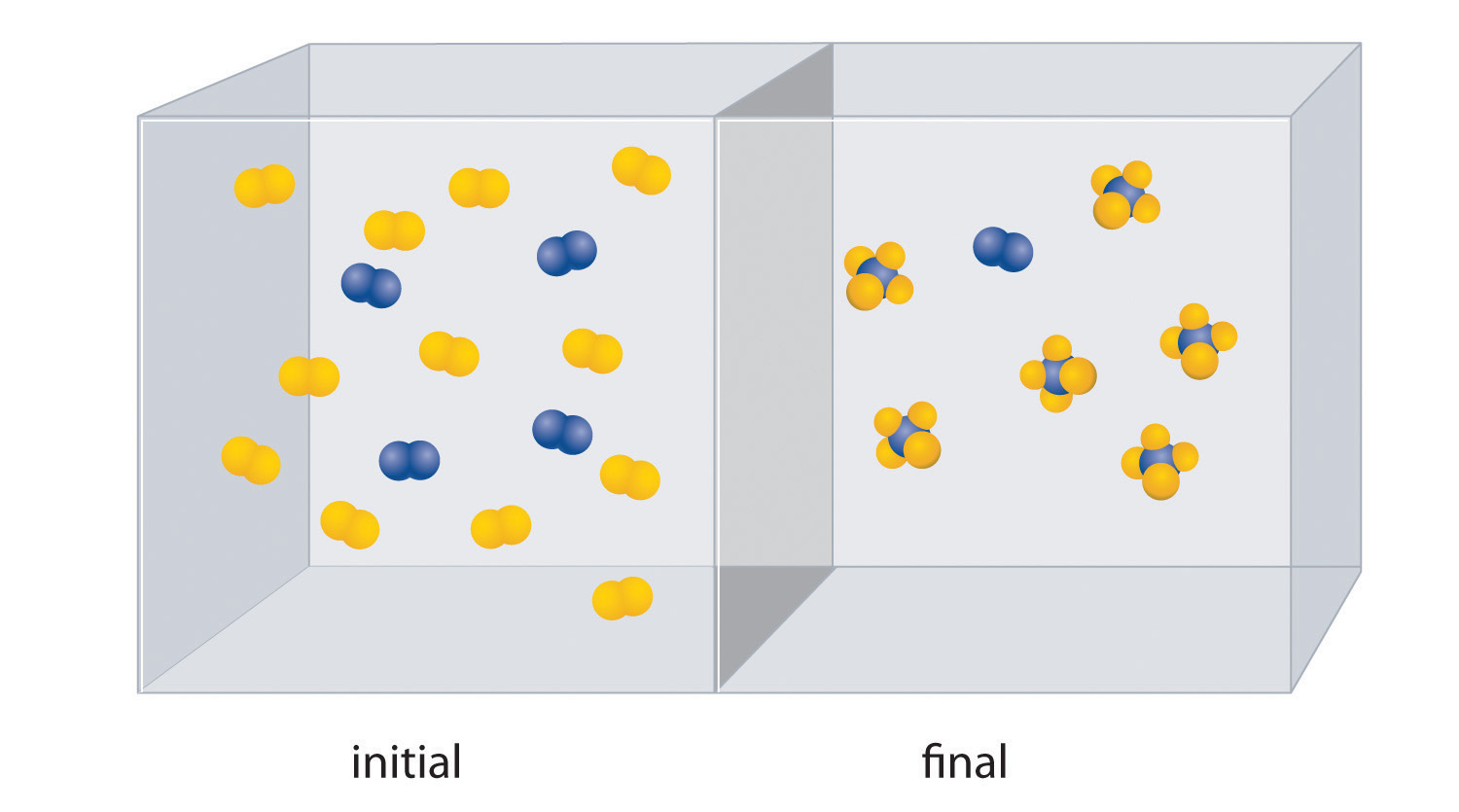1. Write a balanced chemical equation for the reaction.
2. Which is the limiting reactant in the initial reaction mixture?
3. How many moles of the product AB4 could you obtain from a mixture of 0.020 mol A2 and 0.060 mol B2?
15. The reaction of X4 (orange) with Y2 (black) is shown below. The initial reaction mixture is shown on the left and the mixture after the reaction has gone to completion is shown on the right.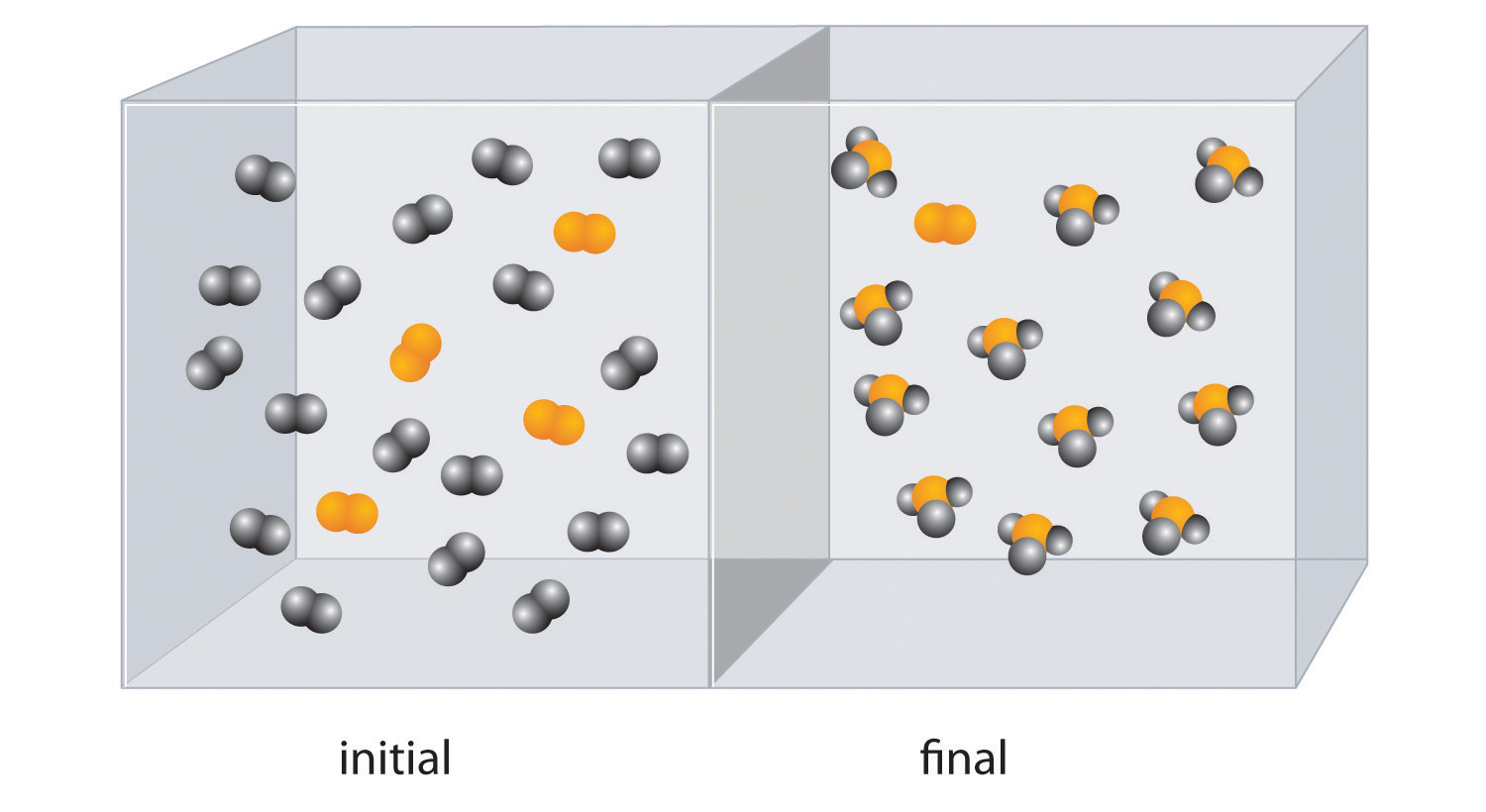1. Write a balanced chemical equation for the reaction.
2. Which is the limiting reactant in the initial reaction mixture?
3. How many moles of the product XY3 could you obtain from a mixture of 0.100 mol X4 and 0.300 mol Y2?
16. Methyl butyrate, an artificial apple flavor used in the food industry, is produced by the reaction of butanoic acid with methanol in the presence of an acid catalyst (H+):

1. Given 7.8 g of butanoic acid, how many grams of methyl butyrate would be synthesized, assuming 100% yield?
2. The reaction produced 5.5 g of methyl butyrate. What was the percent yield?
3. Is the catalyst used in this reaction heterogeneous or homogeneous?
17. In the presence of a platinum catalyst, hydrogen and bromine react at elevated temperatures (300°C) to form hydrogen bromide (heat is indicated by Δ):

Given the following, calculate the mass of hydrogen bromide produced:

1. 8.23 × 1022 molecules of H2
2. 6.1 × 103 mol of H2
3. 1.3 × 105 g of H2
4. Is the catalyst used in this reaction heterogeneous or homogeneous?

### Answers

1. redox reaction
2. exchange
3. acid–base
4. condensation
1. S, −2; N, −3; H, +1
2. P, +5; O, −2
3. F, −1; Al, +3
4. S, −2; Cu, +2
5. H, +1; O, −2; C, +4
6. H, +1; N, −3
7. H, +1; O, −2; S, +6
8. H, +1, O, −2; C, +2
9. butanol:O,−2; H, +1

From left to right: C, −3–2–2–1

1. 2NaHCO3(aq) + H2SO4(aq) → Na2SO4(aq) + 2CO2(g) + 2H2O(l) acid–base reaction

1. Ca, +2; O, −2; C, +4
2. Na, +1; Cl, −1
3. O, −2; C, +4
4. K, +1; O, −2; Cr, +6
5. K, +1; O, −2; Mn, +7
6. O, −2; Fe, +3
7. O, −2; H, +1; Cu, +2
8. O, −2; S, +6
9.

HexanolO,−2; H, +1

From left to right: C: −3, −2, −2, −2, −2, −1

1. Na is the reductant and is oxidized. Cl2 is the oxidant and is reduced.
2. Mg is the reductant and is oxidized. Si is the oxidant and is reduced.
3. H2O2 is both the oxidant and reductant. One molecule is oxidized, and one molecule is reduced.
1. H2O(g) + C(s) $→Δ$ H2(g) + CO(g)

C is the reductant and is oxidized. H2O is the oxidant and is reduced.

2. 8Mn(s) + S8(s) + 8CaO(s) → 8CaS(s) + 8MnO(s)

Mn is the reductant and is oxidized. The S8 is the oxidant and is reduced.

3. 2C2H4(g) + O2(g) $→Δ$ 2C2H4O(g)

Ethylene is the reductant and is oxidized. O2 is the oxidant and is reduced.

4. 8ZnS(s) + 8H2SO4(aq) + 4O2(g) → 8ZnSO4(aq) + S8(s) + 8H2O(l)

Sulfide in ZnS is the reductant and is oxidized. O2 is the oxidant and is reduced.

1. Na2SO4 + 2C + 4NaOH → 2Na2CO3 + Na2S + 2H2O
2. The sulfate ion is the oxidant, and the reductant is carbon.
3. 470 g
4. 3300 g
5. carbon
1. 22.1 g
2. 9.9 × 105 g
3. 1.0 × 107 g
4. heterogeneous